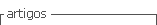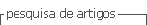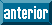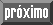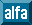## artigo

•Acessos

## versão On-line ISSN 2177-3548

#### Resumo

DALTO, Jader Otavio  e  HAYDU, Verônica Bender. Stimulus equivalence for teaching mathematical first-degree functions in the elementary school. Perspectivas [online]. 2015, vol.6, n.2, pp. 132-146. ISSN 2177-3548.  http://dx.doi.org/10.18761/pac.2015.022.

In this study, it was verified if the stimulus equivalence paradigm is efficient and effective for teaching and learning mathematical first-degree functions. Nine students from the eighth grade of elementary school participated in this study, who underwent written pretest and post-test and a teaching procedure on the computer. The software Equivalence was used for teaching and testing emergence of conditional relations between elements of algebraic language of first-degree functions y=x+1, y=x+2, y=x-1, y=x-2. On the computer, the task was divided in four phases, each one formed by training blocks, emergent relations´ testes and stimuli generalization. After the tests, the emergence of relations between tables, expressions and graphs of the functions y=x, y=x-3, y=x+4 and y=x+3 (stimuli generalization) was assessed. Eight of nine participants formed equivalence classes and seven of the nine participants presented stimuli generalization. These results, consistent with the results presented in literature, show stimulus equivalence paradigm as an efficient and effective strategy for teaching and learning Mathematics.

Palavras-chave : Mathematics Education; Stimulus Equivalence; First-degree function.

· resumo em Português | Espanhol     · texto em Português     · Português (pdf )